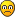zekageri
Posts: 35
Joined: Mon Sep 03, 2018 11:04 am

Is anybody used an external adc with esp32?
I want to use the MCP33131D SPI adc.
https://www.microchip.com/wwwproducts/en/MCP33131D-10
Any toughts?

idahowalker
Posts: 132
Joined: Wed Aug 01, 2018 12:06 pm

How is it better then
The ESP32 integrates two 12-bit SAR (Successive Approximation Register) ADCs supporting a total of 18 measurement channels (analog enabled pins).
?

zekageri
Posts: 35
Joined: Mon Sep 03, 2018 11:04 am

ESP's internal ADC is crap in itself.
I can not measure properly in it.
I was used the adc1 with i2s dma before, and the signal was noisy A.F.
I realized that i need to pull the gpio36 and 39 down because of the noise.
So i lost two pins because of just that.
After that i realized that if i get any interrupt on any of the ADC1 pins, the i2s goes crazy.
So if i want to read analog values with the internal adc i have to use all the channels just for that.
This is not satisfying at all.

idahowalker
Posts: 132
Joined: Wed Aug 01, 2018 12:06 pm

I get great results from the built in A:D's

and an example of the way I am using the ADC.

Code: Select all

``````#include <driver/adc.h>

setup()
{
// set up A:D channels
/*
When VDD_A is 3.3V: 11dB attenuation (ADC_ATTEN_11db) gives full-scale voltage 3.3V
*/
}
////
{
// String localBuffer;
// localBuffer.reserve ( StringBufferSize300 );
int iBit = 1;
float offSET = 1.0f;
float r1 = 100800.0f; // R1 in ohm, 100k = 100,800.0 //
float r2 = 38780.0f; // R2 in ohm, 38k = 38000.0 //actual 38780K
float uPvolts = 3.3f;
// ADC1 channel 0 is GPIO36
// ADC1 channel 1 is GPIO37
// ADC1 channel 6 is GPIO34
// to get resistor R2 go to:
// http://www.ohmslawcalculator.com/voltage-divider-calculator
//   used 12 volts for the input voltage to calculate R2, used 100K for R1
for (;;)
{
// group handle, WaitForBits, ClearOnExitBit, WaitForAllBits, TicksToWait
iBit = iBit << 1;
//    //    Serial.println ( iBit );
if ( iBit == 1073741824 )
{
if ( xSemaphoreTake( sema_AnalogVoltRead_LIDAR, xSemaphoreTicksToWait ) == pdTRUE )
{
ptrVbatt_LIDAR += ( ((( uPvolts * adc1_get_raw(ADC1_CHANNEL_6)) / ADbits) / r2 * ( r1 + r2)) + offSET );
ptrVbatt_LIDAR = ptrVbatt_LIDAR / 2; // average readings
} // if ( xSemaphoreTake( sema_AnalogVoltRead_LIDAR, xSemaphoreTicksToWait ) == pdTRUE )
iBit = 1;
} // if ( iBit == 1073741824 )
}
}
``````

knightridar
Posts: 15
Joined: Sun Nov 25, 2018 10:05 pm

It takes values from an array and smooths them out.
viewtopic.php?f=18&t=13053

I get good results after smoothing out the values and using 0.1 uF capacitors.
See this in Espressif documentation:

Multisampling and capacitors help a lot.

Idahowalker's advice that I got a while back about using the code in post I mentioned above is also good versus using analogRead commands of Arduino IDE.
AttachmentsPhotodiodes ADC values with .1 uF capacitors.jpg (2.43 MiB) Viewed 309 times

idahowalker
Posts: 132
Joined: Wed Aug 01, 2018 12:06 pm

Also, I found that by sampling the ADC regularly once a mS or so, and applying the Simple Kalman filter to the results https://github.com/denyssene/SimpleKalmanFilter, the output gets real smooth. Even if the A:D converter values are not displayed regularly, like once a second, getting several readings per second and apply the simple Kalman with each sampling does a real good job.

The simple Kalman results are better with an accurate time between iterations.

Here

Code: Select all

``SimpleKalmanFilter X_KF( (float)iX_Posit90, (float)iX_Posit90, float(ServoDelay12mS) / 1000.0f );``
I use the time of task iteration.

Here I use the 64 bit ESP32 uS clock to get time iterations.

Code: Select all

``````void fDo_AudioReadFreq( void *pvParameters )
{
int64_t EndTime = esp_timer_get_time();
int64_t StartTime = esp_timer_get_time(); //gets time in uSeconds like Arduino Micros
for (;;)
{
xEventGroupWaitBits (eg, evtDo_AudioReadFreq, pdTRUE, pdTRUE, portMAX_DELAY);
EndTime = esp_timer_get_time() - StartTime;
// the other code stuff thing do's
// and more code thing do's
// and even more code thing do's
StartTime = esp_timer_get_time();
// }
}
} // fDo_ AudioReadFreq( void *pvParameters )``````

zekageri
Posts: 35
Joined: Mon Sep 03, 2018 11:04 am

I was using the adc with i2s dma. My code looks like this:

I2S config:

Code: Select all

``````void configure_i2s(){
//pinMode(36,INPUT);
i2s_config_t i2s_config =
{
.sample_rate = 144444,                                             // sample rate
.bits_per_sample = I2S_BITS_PER_SAMPLE_16BIT,                                 // 16 bit I2S
.channel_format = I2S_CHANNEL_FMT_ONLY_LEFT, //I2S_CHANNEL_FMT_ALL_LEFT,                                   // only the left channel
.communication_format = (i2s_comm_format_t)(I2S_COMM_FORMAT_I2S | I2S_COMM_FORMAT_I2S_MSB),   // I2S format
.intr_alloc_flags = 0,                                                        // none
.dma_buf_count = 2,                                                           // number of DMA buffers
.dma_buf_len = 1024,                                                   // number of samples
.use_apll = 0,                                                                // no Audio PLL
};
i2s_driver_install(I2S_NUM_0, &i2s_config, 0, NULL);

}``````
Read from DMA BUFFER and plot the results to web:

Code: Select all

``````static const inline void Plot_DMA_Buffer(){
int Vmax = 0;
int Vmin = 4095;
uint16_t Buffer[NUM_SAMPLES];
for(int i = 0;i<NUM_SAMPLES;i++){
if(i != NUM_SAMPLES)
{
}
}
}
}
}
int Vamp = (Vmax) - (Vmin);
if(millis() - Start_Millis >= 1000){
Start_Millis = millis();
Send_Amplitude(Vamp);
if(olvasunk_e){
for(int k = 0;k<NUM_SAMPLES;k++){
if(!olvasunk_e){
break;
}else{
String data = (String)Buffer[k]+"}";
}
}
}
}
}``````

Code: Select all

``````static const inline void Base_Setups_Core1(){
Serial.begin(115200);
SPIFFS.begin() ? Serial.println("SPIFFS.OK") : Serial.println("SPIFFS.FAIL");
configure_i2s();

/** Ethernet wifi stack **/
WiFi.onEvent(WiFiEvent);
ETH_begin();
ethernet.config(ip1, nm1, gw1);
TCP_Requests();
server.begin();
ws.onEvent(onWsEvent);
External_Interrupts();
}

static const inline void Main_Loop(){
if(!Ota_is_Started){
Plot_DMA_Buffer();                            // RETRIVE DATA FROM i2s_read_buff FOR CALCULATION AND VISUALIZATION / COMPUTE THE AVERAGE AMPLITUDE
}
}

static void loop0(void * pvParameters){
for( ;; ){
I don't know how the task semaphores or the task events are working.